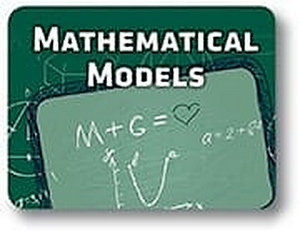Red Comet## Course Details:

This course focuses on modeling with mathematics and problem-solving. Students conduct and analyze research and study the applications of mathematical modeling in finance, purchases, and investments. Students understand models and how to use them to solve real-life problems. Students learn about patterns and transformations and use music as a field of example to study this concept.

## Syllabus:

Unit 6 – Finance

Objectives:

• Determine salary.
• Evaluate and determine the value of other types of compensation, such as benefits.
• Know how to create and calculate budgets.
• Calculate deductions, withholdings, and state taxes using income tax tables.
• Estimate unemployment benefits, such as Social Security and Medicare.
• Calculate sales tax.
• Understand banking terminology.
• Understand how to write checks and balance a checkbook.
• Examine the differences between simple and compound interest, and know how to calculate both.
• Write equations and solve problems involving investments.

Lessons:

• Salary
• Other Forms of Compensation
• Budgeting
• Deductions, Withholdings, State Taxes
• Unemployment Insurance and Social Security
• Sales Tax
• Checking Accounts
• Savings Accounts and Simple Interest
• Compound Interest
• Money Applications

Unit 7 – Purchases

Objectives:

• Determine wholesale, retail, and markup prices.
• Estimate the cost of credit cards.
• Calculate installment loan payments and cost.
• Determine rental costs.
• Determine buying costs, including closing costs and property taxes.
• Calculate the cost and interest of different types of mortgages.
• Understand what an amortization schedule is.
• Compare renting property to buying property.
• Understand the terminology involved in automobile purchases.
• Compare and calculate used vehicle costs, new vehicle costs, and leasing costs.
• Understand and calculate cost of ownership.

Lessons:

• Sales Prices
• Credit Cards
• Installment Payments
• Renting Costs
• Type of Mortgages
• Leasing a Vehicle
• The cost of Ownership

Unit 8 – Investments

Objectives:

• Examine savings investment options.
• Calculate simple and compound interest.
• Compare simple, compound, and continuously compounded interest in relation to investments.
• Examine different types of insurance.
• Understand various insurance terminology.
• Calculate the costs and benefits of insurance, including copayments and deductibles.
• Understand investment terminology, including terminology associated with annuities and bonds.
• Calculate stock values.
• Examine annuity and bond investments.
• Calculate retirement funds.
• Examine the risks and benefits of various investments.

Lessons:

• Simple Interest
• Compound Interest
• Life Insurance
• Health Insurance
• Deductibles and Copayments
• Stocks
• Annuities
• Bonds
• Retirement Plans

Unit 9 - Solving Problems Using Models

Objectives:

• Understand growth and decay models.
• Evaluate growth and decay.
• Apply growth and decay models to real-life situations.
• Understand trigonometric functions and ratios, including inverse functions.
• Use trigonometric functions to solve real-life problems.
• Model periodic motion using sine, cosine, and tangent.
• Understand direct, inverse, and joint variation.
• Connect variation to science concepts and laws of physics.
• Evaluate variation problems.

Lessons:

• Growth Models
• Decay Models
• Modeling Real-life Situations
• Trigonometric Ratios
• Inverse Functions and Trigonometric Proportions
• Periodic Motion
• Direct Variation
• Inverse Variation
• Variation in Science
• Joint Variation

Unit 10 – Transformations, Patterns, and Music

Objectives:

• Identify the types of transformations and their properties.
• Transform geometric shapes through translation, rotation, and reflection.
• Determine whether a figure has reflection or rotational symmetry.
• Understand perspective and patterns in art and architecture.
• Determine scale factor.
• Understand mathematical patterns, including the golden ratio and tessellation.
• Identify and understand transformations in music.
• Calculate proportional relationships in music.
• Understand that ratios can indicate consonance or dissonance in music.
• Model harmonic motion.

Lessons:

• Transformations
• Translations
• Rotation
• Reflections
• Symmetry
• Perspective
• Patterns in Art and Architecture
• Transformations in Music
• Proportional Relationships in Music
• Harmonic Music

### Accreditation & Approvals